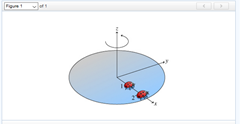# Problem: Two ladybugs sit on a rotating disk, as shown in the figure (the ladybugs are at rest with respect to the surface of the disk and do not slip). (Figure 1) Ladybug 1 is halfway between ladybug 2 and the axis of rotation.What is the ratio of the linear speed of ladybug 2 to that of ladybug 1?

###### FREE Expert Solution

Linear velocity:

$\overline{){\mathbf{v}}{\mathbf{=}}{\mathbf{r}}{\mathbf{\omega }}}$

91% (492 ratings)###### Problem Details

Two ladybugs sit on a rotating disk, as shown in the figure (the ladybugs are at rest with respect to the surface of the disk and do not slip). (Figure 1) Ladybug 1 is halfway between ladybug 2 and the axis of rotation.What is the ratio of the linear speed of ladybug 2 to that of ladybug 1?